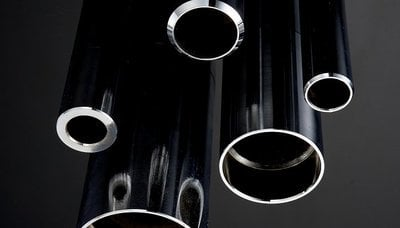# Make Sense of Calculations for Tube Bending with Our Tube Bending Calculator

October 18, 2023 by
Mike Thomas

Performing your calculations for tube bending before you bend is a necessary, and sometimes tedious, part of the bending process. We've created a tool to help you navigate the bending calculations you're likely to need, but it's also important to understand why your calculations need to be correct.

## What you need to know to perform tube bending

There are a couple of pieces of important information you will need to calculate before you begin bending.

To perform a bend, the tube bender must have enough bend arm torque to overcome a material's section modulus. If the bender does not have enough torque, the bend will fail to be permanent, or the material will be distorted or deformed. This is why calculating the section modulus as well as the torque is so vital for performing bends correctly.

The material composition is also important because it determines the deforming of the tubing material past the given material's modulus of elasticity and into the yield strength of the material.

Some of the information you should know before you attempt your calculations for tube bending include the tubes outside diameter, the wall thickness, and the centerline radius. Of course, this is only a partial list so it's important to refer to the formulas you will be using before beginning your calculations.

All in all, there are many variables that go into performing a successful tube bend. The calculations for CNC tube benders get even more tricky when dealing with non-round shapes.

## Can you bend non-round tubes?

Yes. To understand how your bender will bend square, rectangular, and other non- round shapes you first need to calculate the section area moment of inertia for the round material and then compare it to the non-round material. As long as the round material number is higher than the non-round material, then that particular bender will be capable of bending your part shape.

It can be confusing to know which formulas and information you'll need to perform these calculations, which is where our tube bending section modulus calculator can help.

## What is the tube bending section modulus calculator?

The tube bending section modulus calculator allows you to input the tube specifications into an easy to use calculator. It then calculates the section area moment of inertia properties of common shapes.Some of the most common shapes covered by the calculator are:

• Square
• Round
• Half rounds
• Triangles
• Rectangles
• Trapezoids
• Hexagons
• Octagons

## What is in the Guide?

The Tube Bending Calculator Guide that is bundled with the calculator covers some of the most common tube bending formulas, including:

• Tube inside diameter
• Wall factor